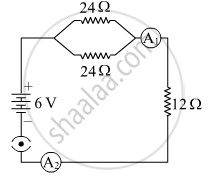# Study the following circuit and find out: (i) Current in 12 Ω resistor. (ii) The difference in the readings of A1 and A2, if any. - Science

Diagram

Study the following circuit and find out:
(i) Current in 12 Ω resistor.
(ii) The difference in the readings of A1 and A2, if any.#### SolutionFirstly calculating the equivalent resistance in the circuit.

"R"_"eq" =( 24xx24)/(24+24)+12=24Ω

(i) The current through the 12 Ω resistor is given by:

"I" = 6/24=1/4 "A"

(ii) The difference in the readings of A1 and Ais zero, as they are in series connected with the same wire.
Is there an error in this question or solution?
2018-2019 (March) 31/4/2

Share